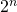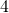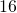# R Functions for Best Subset RegressionBest subset regression is an technique for model building and variable selection. The method looks at all combinations of independent predictor variables for use in a multiple regression model. Model developers and analysts will often struggle with variable selection, especially when the number of predictors is high.  Ideally, each set of predictors is run and the best set is selected using a criteria for model performance. The following article provides custom functions for best subset selection that are fast and easy to use.

#### Method Steps and Challenges

Best subset regression has three basic steps:

• Define predictor variables and the model grid of predictor combinations;
• Run models for all combinations of predictors;
• Apply criteria to select the model with the best subset of predictors.

The challenge in coding the method is to two-fold:

• Efficiency: As the number of predictors increase, the number of combinations increases at the rate of.  The use of loops for model runs and performance assessment are inefficient and can be avoided using vectorized functions in R;
• Selection Bias: The model selection criteria is also important.  Some criteria are prone to bias (like p-value significant testing) when regression model assumptions are no longer valid.

#### The Model Grid of Predictor Combinations

The model grid can be found using the function below:

For example, withpredictor variables, we getorcombinations, as shown below. A value ofindicates the model predictor is on and a value of zero indicates the predictor is off:

#### Best Subset Regression – Model runs and Diagnostics

Next, another function is provide that will run all model combinations and diagnostics.

The function above requires 4 installed packages. You’ll see there is no conditional looping  used in the code.  Instead, the vectorized map() function from the purrr package is used. At the same time, the %>% operator from the magrittr package is used. The forecast package is also called to use the cross-validation function CV(), which has the 5 metrics or selection criteria to rank the predictor subsets. Finally, the dplyr package is used to arrange the function results.  Note that the tslm() model function is specified in this example…others could be used such as vglm(). Simply swap in the model function you want.

#### Function Testing Example

Here is an application example of the best.subset() function.  A simple model and data set  is used from the book Forecasting Principles and Practice by Robert Hyndman and Geoge Athanasopoulos.  In this case, parameter selection is required to explain US consumption.  The potential predictors  include national income, production, unemployment and savings.  The example uses the uschange data from the same book, which is found in the fpp2 package.

Only 15 predictor combinations are profiled in the output above since the model combination with all predictors turned off has been dropped.  The model grid of predictors is presented in the first 4 columns of the results table.  Next, the table ranks the different model fits using 5 criteria from the CV() function.  The “best” combination of predictors is the subset that minimizes the value of the metrics (CV, AIC, AICc, and BIC) and maximizes adjusted R-squared.1

Looking at the table, the best subset is the one with all predictors on at th etop of the list. However, the second row uses only 3 of 4 variables and the performance results are roughly the same. Also note that after row 4, the model results begin to degrade. Thats because income and savings appear to be the key drivers of consumption. As these two variables are dropped from the predictors, model performance drops significantly.

The performance of the custom function is solid since the results presented here match those of the book referenced.

1. See the forecast package for a more detailed description of the model performance metrics used.
This entry was posted in Data Science, Faster R, Modeling. Bookmark the permalink.